# Linked Representation list elements are stored in memory

• Slides: 20Linked Representation • list elements are stored, in memory, in an arbitrary order • explicit information (called a link) is used to go from one element to the nextMemory Layout of L = (a, b, c, d, e) using an array representation. a b c d e A linked representation uses an arbitrary layout. c a e d b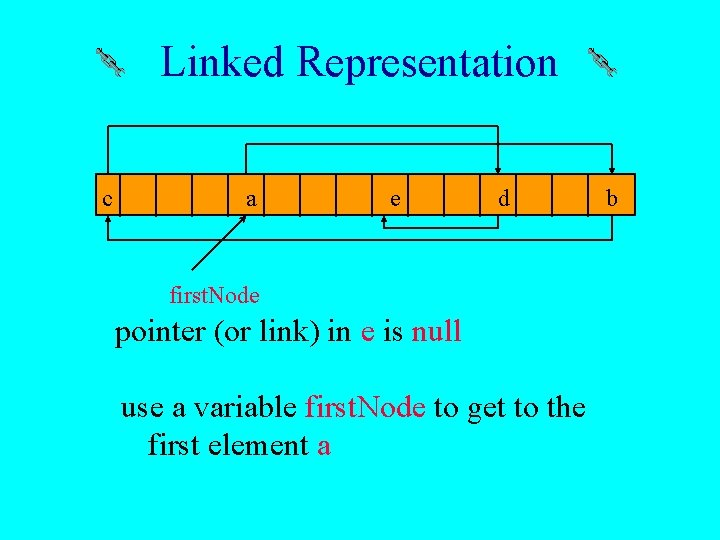Linked Representation c a e d first. Node pointer (or link) in e is null use a variable first. Node to get to the first element a bNormal Way To Draw A Linked List first. Node null a b c link or pointer field of node data field of node d e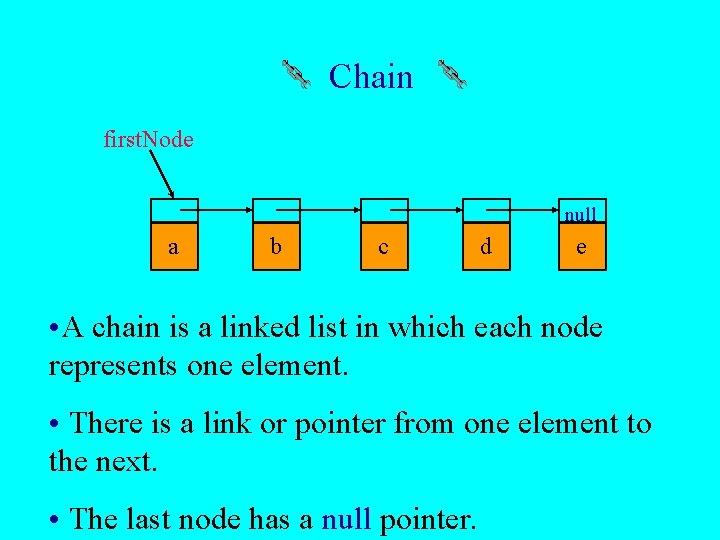Chain first. Node null a b c d e • A chain is a linked list in which each node represents one element. • There is a link or pointer from one element to the next. • The last node has a null pointer.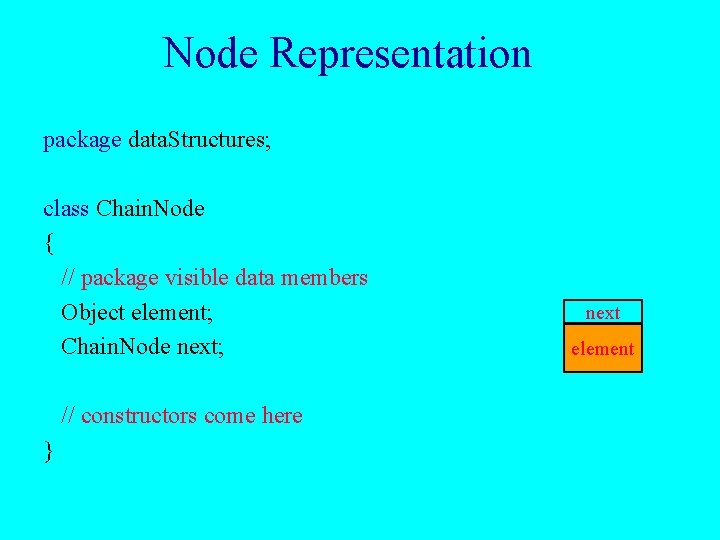Node Representation package data. Structures; class Chain. Node { // package visible data members Object element; Chain. Node next; // constructors come here } next element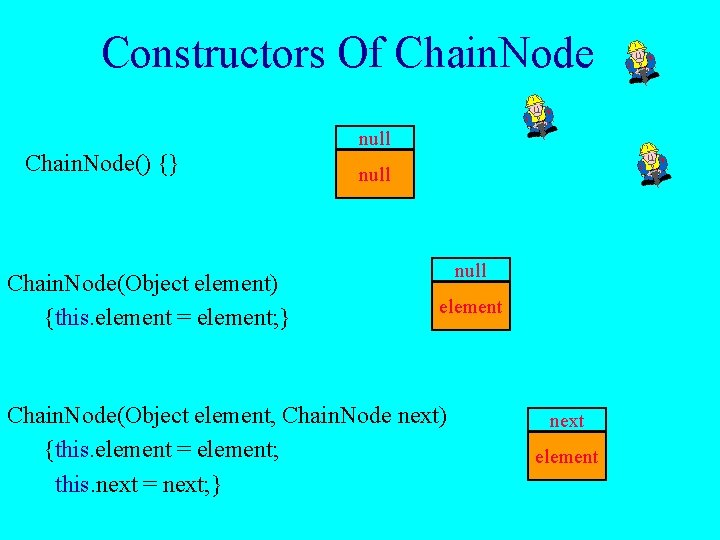Constructors Of Chain. Node null Chain. Node() {} Chain. Node(Object element) {this. element = element; } null element Chain. Node(Object element, Chain. Node next) {this. element = element; this. next = next; } next elementget(0) first. Node null a b c d e desired. Node = first. Node; // gets you to first nodeget(1) first. Node null a b c desired. Node = first. Node. next; // gets you to second node d e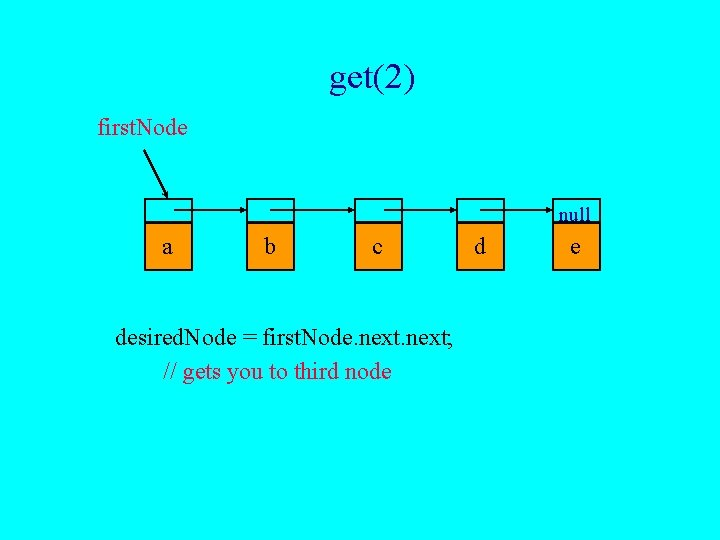get(2) first. Node null a b c desired. Node = first. Node. next; // gets you to third node d e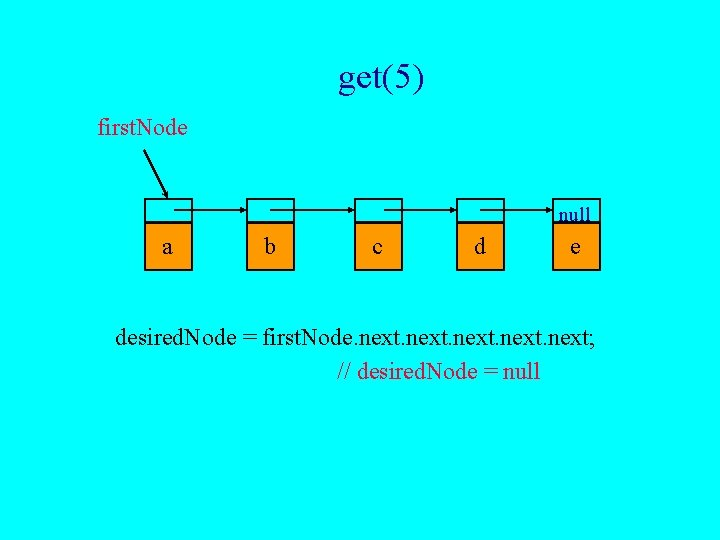get(5) first. Node null a b c d e desired. Node = first. Node. next; // desired. Node = null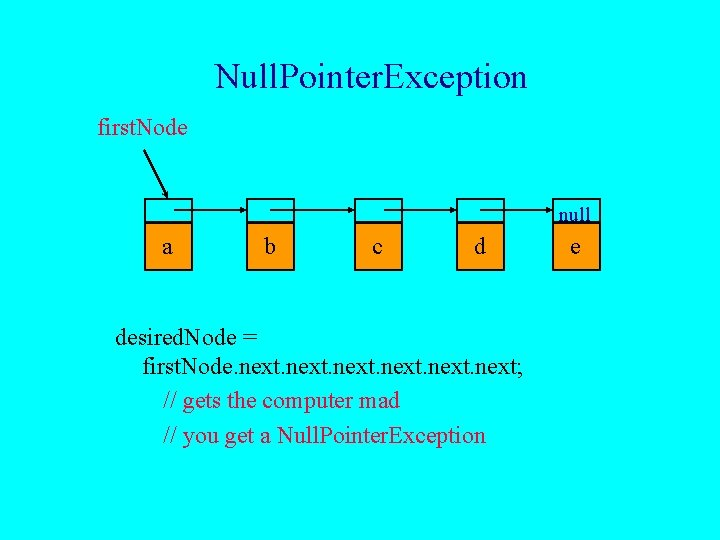Null. Pointer. Exception first. Node null a b c d desired. Node = first. Node. next; // gets the computer mad // you get a Null. Pointer. Exception e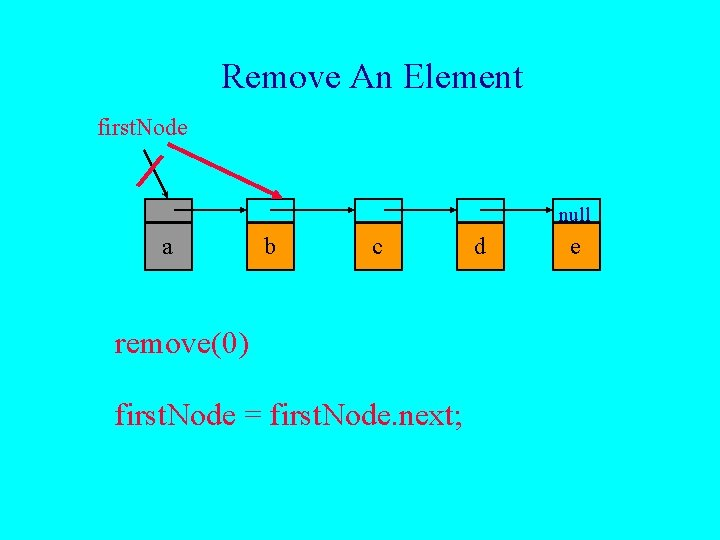Remove An Element first. Node null a b c remove(0) first. Node = first. Node. next; d eremove(2) first. Node a b c null c d before. Node e first get to node just before node to be removed before. Node = first. Node. next;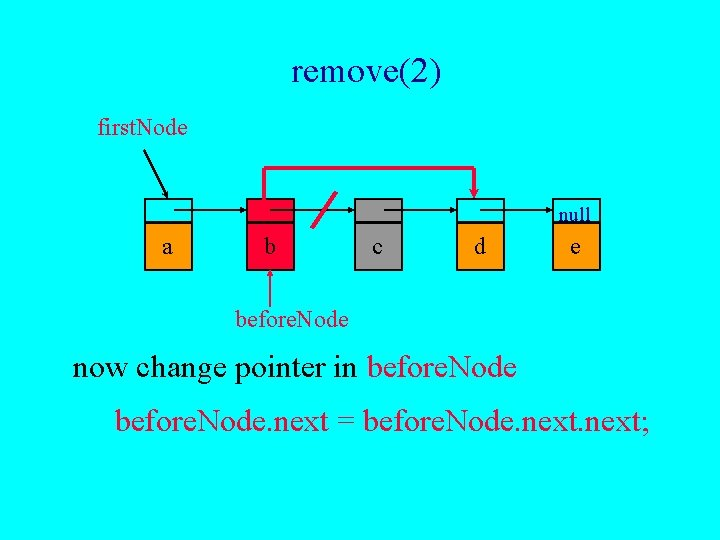remove(2) first. Node null a b c d e before. Node now change pointer in before. Node. next = before. Node. next;add(0, ’f’) first. Node null f a b c d e new. Node Step 1: get a node, set its data and link fields Chain. Node new. Node = new Chain. Node(new Character(‘f’), first. Node);add(0, ’f’) first. Node null f a b c new. Node Step 2: update first. Node = new. Node; d e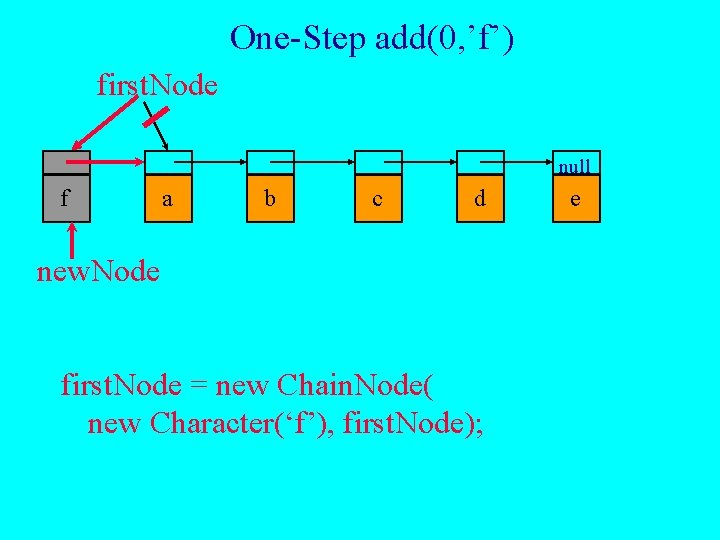One-Step add(0, ’f’) first. Node null f a b c d new. Node first. Node = new Chain. Node( new Character(‘f’), first. Node); eadd(3, ’f’) first. Node new. Node f null a b c d e before. Node • first find node whose index is 2 • next create a node and set its data and link fields Chain. Node new. Node = new Chain. Node(new Character(‘f’), before. Node. next); • finally link before. Node to new. Node before. Node. next = new. Node;Two-Step add(3, ’f’) first. Node new. Node f null a b c d e before. Node = first. Node. next; before. Node. next = new Chain. Node(new Character(‘f’), before. Node. next);